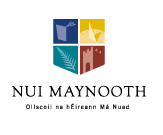Irish Geometry Conference 2013 Dept of Mathematics and Statistics, NUI Maynooth 20–21 June 2013

## Titles and abstracts

John Burns, NUI Galway
The geometry of Weyl groups and their maximal tori
Motivated by a question from Theoretical Physics, we classify the conjugacy classes of maximal order Abelian subgroups of Weyl groups and establish a version of Cartan's theorem on the conjugacy of maximal tori in this context. The classification and applications will encounter many old friends and some new ones (Symmetric spaces, branching rules, Del Pezzo surfaces, Fano planes and qubits).

Diarmuid Crowley, Max Planck Institute for Mathematics
A new invariant of $G_2$ structures
I will report on recent work where we define an invariant of diffeomorphisms and homotopies of a $G_2$ structure on a closed 7-manifold $M$. The $\nu$-invariant takes values in $\Bbb Z/48$ and is defined via the Euler characteristic and signature of a $\text{Spin}(7)$-coboundary of the $G_2$ structure.
An important motivation for defining the $\nu$-invariant is to investigate the connectivity of moduli space of $G_2$-metrics on $M$ in the case where such metrics exist.
I will discuss examples, calculations for the invariant to date and its relationship to the mapping class groups of spin 7-manifolds. This work is joint with Johannes Nordström.

Emily Dryden, Bucknell University
Beating and heating planar domains
If you had perfect pitch and listened to a recording of the sounds a drum made when struck, could you determine the shape of the drum? Phrased more mathematically, do the eigenvalues of the Dirichlet Laplace operator acting on smooth functions on a piecewise smooth planar domain $D$ determine $D$ up to isometry? This question was answered in the negative in 1992 by Carolyn Gordon, David Webb, and Scott Wolpert. Their answer tells us that these eigenvalues are an incomplete set of geometric invariants, and it is natural to look for ways to distinguish such non-isometric sound-alike drums. After introducing some examples of drums that sound alike, we will examine what we can learn from heating these drums and studying the amount of heat in them over time. This is joint work with Michiel van den Berg and Thomas Kappeler.

Thomas Huettemann, Queen's University Belfast
On Novikov cohomology of cochain complexes
Informally, Novikov cohomology of a cochain complex is the cohomology of a "completion" of the cochain complex. I will discuss the case of a cochain complex over a Laurent polynomial ring in finitely many indeterminates, in which case Novikov cohomology detects certain finiteness properties of the original cochain complex. Concrete results proved in collaboration with David Quinn make heavy use of a toric re-interpretation of a known one-variable result due to Ranicki. I will explain the relevant constructions in some detail, concentrating on basic ideas and geometric input.

Ben McKay, University College Cork
Holomorphic geometry on compact complex manifolds
I will explain some of the techniques that went into the classification of various types of holomorphic geometric structure (2nd order ODEs in particular) on compact Kaehler manifolds.

Niall Ó Murchadha, University College Cork
Two scalar functions define all axi-symmetric transverse tracefree tensors on flat space
A TT tensor is a symmetric $3 \times 3$ matrix for which each row is divergence-free and the tensor is also tracefree. This means that one has 6 independent components satisfying 4 equations. Therefore one expects 2 degrees of freedom per space point. Such TT tensors arise naturally in several areas in General Relativity. I will show that all axisymmetric TT tensors on flat space can be constructed from 2 axi-symmetric scalar potentials.

Wilderich Tuschmann, Karlsruhe Institute of Technology
Manifolds with almost nonnegative curvature operator
The curvature tensor of a Riemannian manifold also gives rise to a symmetric operator on two-forms, the curvature operator. Closed manifolds admitting metrics with almost nonnegative curvature operator play an important role in the emerging theory of collapsing under a lower bound on the curvature operator. I will discuss constructions of spaces with as well as global obstructions to this property.

Mark Walsh, Wichita State University
Loop Spaces and Positive Scalar Curvature
Recently, there have been a number of interesting developments concerning the problem of understanding the space of metrics of positive scalar curvature on a smooth manifold. In this talk, I will first provide some background to this problem. I will then discuss a new result which shows that in the case when the underlying manifold is a sphere of dimension $n$, where $n$ is at least three but not equal to four, the space of metrics of positive scalar curvature is weakly homotopy equivalent to an $n$-fold loop space. This result makes considerable use of a recent theorem of Botvinnik on concordance and isotopy in the space of metrics of positive scalar curvature.

Colum Watt, Dublin Institute of Technology
Milnor fibres and non-crossing partitions
Each arrangement of hyperplanes in a complex vector space has an associated fibration whose base space is the once-punctured complex plane and whose fibre is called the Milnor fibre. In this talk, non-crossing partitions are used to describe a new triangulated space which can be used to shed new light on the Milnor fibre when the arrangement arises from a finite reflection group.
This is joint work with Thomas Brady and Michael Falk (Northern Arizona University).